Courses

# Relations for ΔH and ΔS for Liquids Civil Engineering (CE) Notes | EduRev

## Civil Engineering (CE) : Relations for ΔH and ΔS for Liquids Civil Engineering (CE) Notes | EduRev

The document Relations for ΔH and ΔS for Liquids Civil Engineering (CE) Notes | EduRev is a part of the Civil Engineering (CE) Course Thermodynamics.
All you need of Civil Engineering (CE) at this link: Civil Engineering (CE)

Relations for ΔH and ΔS for Liquids

One starts with the generic equations for dH and dS developed in section 5.4.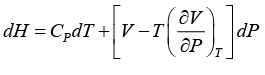.......(5.20)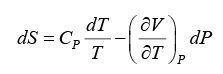.......(5.22)

As discussed in section 2.3, for liquids it is often simpler to use volume expansivity and isothermal compressibility parameters for computing thermodynamic properties of interest.  Thus from eqn. 2.3: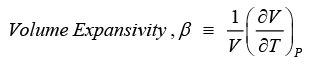Using eqn. 2.3 in 5.20 and 5.22 the following relations obtain: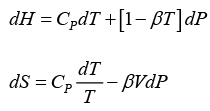.......(5.66, 5.67)

The above equations may also be used to compute the properties of a compressed liquid state. Since the volumetric properties of liquids are very weakly dependent of pressure one can often use the saturated liquid phase properties as reference points and integrate the eqns. 5.66 to 5.67 (at constant temperature) to obtain enthalpy and entropy respectively. The relevant equations are: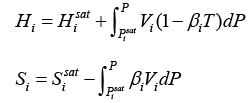.......(5.68, 5.69)

In the last two equations, the molar volume V i may be set equal to Vi sat (liq.) , and the volume expansivity approximated to that at the saturated liquid point at the given temperature.

Offer running on EduRev: Apply code STAYHOME200 to get INR 200 off on our premium plan EduRev Infinity!

## Thermodynamics

56 videos|92 docs|33 tests

,

,

,

,

,

,

,

,

,

,

,

,

,

,

,

,

,

,

,

,

,

;